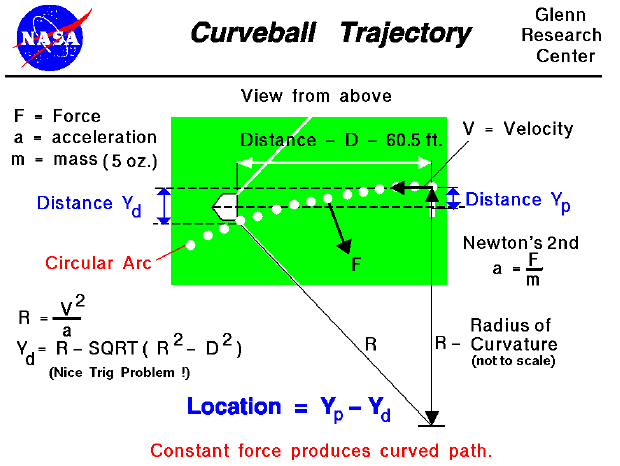All that is necessary to create lift is to turn a flow of air. An airfoil of a wing will turn a flow, but so will a spinning baseball. The details of how the force is generated are fairly complex, but the magnitude of the force (F) depends on the radius of the ball (b), the spin of the ball (G), the velocity (V) of the pitch, and the density (r) of the air.

F = r * G * V * b * pi / 2

As the force acts on the ball, it will be deflected along it's flight path. If we neglect the viscous forces on the ball (which will slow it down and change the magnitude and direction of the force), we have a constant force always acting perpendicular (at a right angle) to the flow direction. The resulting flight path is a circular arc. On the figure, we see the trajectory of the baseball as it moves from the pitching mound to the plate (right to left). Each white dot is the location of the ball at .05 second intervals (it gets to the plate pretty fast!). The radius of curvature (R) of the flight path depends on the velocity (V) of the pitch and the acceleration (a) produced by the force.

R = V ^2 / a

We can solve for this acceleration from Newton's second law of motion using the force for a spinning ball and the mass (m) of the baseball (5 oz.).

a = F / m

Since the radius of curvature depends on the force, all the factors that affect the force will also affect the trajectory. The higher the spin, the sharper the curve; the higher the velocity, the straighter the curve. At higher altitudes, the density is lower and the curve will straighten out. (It's very hard to make a ball curve on Mt. Everest, no matter how much it spins.)

Knowing the radius of curvature and the distance from the pitching mound to home plate, we can also calculate the distance that the ball is deflected (Yd) along the flight path. The pitcher can release the ball at different distances from the center of the plate (Yp). The difference between Yp and Yd will give the final location of the pitch relative to the center of the plate.

You can investigate the effect of aerodynamics on throwing a curve ball by using the CurveBall Java Applet. Have fun ! You can use the browser "Back" button to return to this page. If want your own copy of CurveBall to play with, you can download it for free.

Guided Tours

Back to top

Go to...

Beginner's Guide Home Page

byTom Benson
Please send suggestions/corrections to: benson@grc.nasa.gov